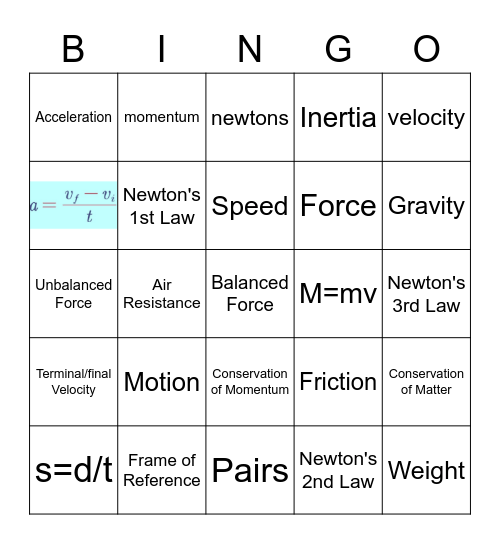# Motion Bingo IIThis bingo card has 1 images, a free space and 23 words: Motion, Frame of Reference, Speed, s=d/t, velocity, Acceleration, momentum, M=mv, Conservation of Momentum, Force, Unbalanced Force, Friction, Conservation of Matter, Newton's 1st Law, Newton's 2nd Law, Newton's 3rd Law, newtons, Gravity, Air Resistance, Weight, Terminal/final Velocity, Inertia and Pairs.

## Play Online